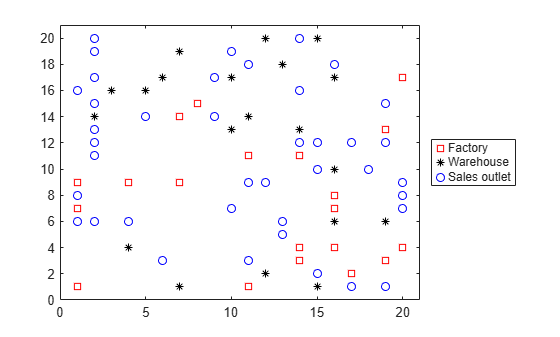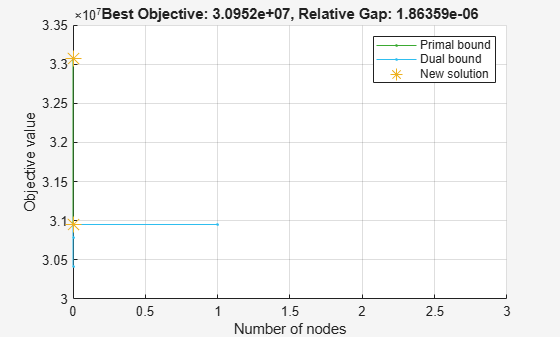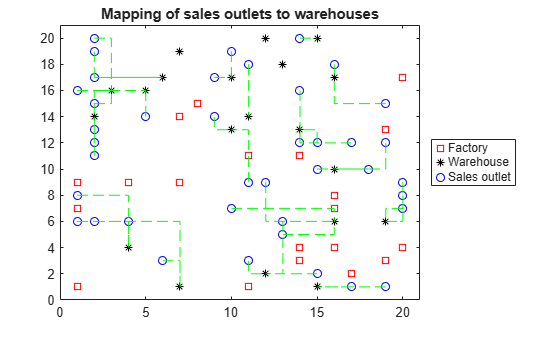# Factory, Warehouse, Sales Allocation Model: Solver-Based

This example shows how to set up and solve a mixed-integer linear programming problem. The problem is to find the optimal production and distribution levels among a set of factories, warehouses, and sales outlets. For the problem-based approach, see Factory, Warehouse, Sales Allocation Model: Problem-Based.

The example first generates random locations for factories, warehouses, and sales outlets. Feel free to modify the scaling parameter $N$, which scales both the size of the grid in which the production and distribution facilities reside, but also scales the number of these facilities so that the density of facilities of each type per grid area is independent of $N$.

### Facility Locations

For a given value of the scaling parameter $N$, suppose that there are the following:

• $⌊f{N}^{2}⌋$ factories

• $⌊w{N}^{2}⌋$ warehouses

• $⌊s{N}^{2}⌋$ sales outlets

These facilities are on separate integer grid points between 1 and $N$ in the $x$ and $y$ directions. In order that the facilities have separate locations, you require that $f+w+s\le 1$. In this example, take $N=20$, $f=0.05$, $w=0.05$, and $s=0.1$.

### Production and Distribution

There are $P$ products made by the factories. Take $P=20$.

The demand for each product $p$ in a sales outlet $s$ is $d\left(s,p\right)$. The demand is the quantity that can be sold in a time interval. One constraint on the model is that the demand is met, meaning the system produces and distributes exactly the quantities in the demand.

There are capacity constraints on each factory and each warehouse.

• The production of product $p$ at factory $f$ is less than $pcap\left(f,p\right)$.

• The capacity of warehouse $w$ is $wcap\left(w\right)$.

• The amount of product $p$ that can be transported from warehouse $w$ to a sales outlet in the time interval is less than $turn\left(p\right)*wcap\left(w\right)$, where $turn\left(p\right)$ is the turnover rate of product $p$.

Suppose that each sales outlet receives its supplies from just one warehouse. Part of the problem is to determine the cheapest mapping of sales outlets to warehouses.

### Costs

The cost of transporting products from factory to warehouse, and from warehouse to sales outlet, depends on the distance between the facilities, and on the particular product. If $dist\left(a,b\right)$ is the distance between facilities $a$ and $b$, then the cost of shipping a product $p$ between these facilities is the distance times the transportation cost $tcost\left(p\right)$:

`$dist\left(a,b\right)*tcost\left(p\right).$`

The distance in this example is the grid distance, also known as the ${L}_{1}$ distance. It is the sum of the absolute difference in $x$ coordinates and $y$ coordinates.

The cost of making a unit of product $p$ in factory $f$ is $pcost\left(f,p\right)$.

### Optimization Problem

Given a set of facility locations, and the demands and capacity constraints, find:

• A production level of each product at each factory

• A distribution schedule for products from factories to warehouses

• A distribution schedule for products from warehouses to sales outlets

These quantities must ensure that demand is satisfied and total cost is minimized. Also, each sales outlet is required to receive all its products from exactly one warehouse.

### Variables and Equations for the Optimization Problem

The control variables, meaning the ones you can change in the optimization, are

• $x\left(p,f,w\right)$ = the amount of product $p$ that is transported from factory $f$ to warehouse $w$

• $y\left(s,w\right)$ = a binary variable taking value 1 when sales outlet $s$ is associated with warehouse $w$

The objective function to minimize is

`$\sum _{f}\sum _{p}\sum _{w}x\left(p,f,w\right)\phantom{\rule{0.5em}{0ex}}\cdot \phantom{\rule{0.5em}{0ex}}\left(pcost\left(f,p\right)+tcost\left(p\right)\cdot dist\left(f,w\right)\right)$`

`$+\sum _{s}\sum _{w}\sum _{p}\left(d\left(s,p\right)\phantom{\rule{0.5em}{0ex}}\cdot \phantom{\rule{0.5em}{0ex}}tcost\left(p\right)\phantom{\rule{0.5em}{0ex}}\cdot \phantom{\rule{0.5em}{0ex}}dist\left(s,w\right)\phantom{\rule{0.5em}{0ex}}\cdot \phantom{\rule{0.5em}{0ex}}y\left(s,w\right)\right).$`

The constraints are

$\sum _{w}x\left(p,f,w\right)\le pcap\left(f,p\right)$ (capacity of factory).

$\sum _{f}x\left(p,f,w\right)=\sum _{s}\left(d\left(s,p\right)\phantom{\rule{0.5em}{0ex}}\cdot \phantom{\rule{0.5em}{0ex}}y\left(s,w\right)\right)$ (demand is met).

$\sum _{p}\sum _{s}\frac{d\left(s,p\right)}{turn\left(p\right)}\phantom{\rule{0.5em}{0ex}}\cdot \phantom{\rule{0.5em}{0ex}}y\left(s,w\right)\le wcap\left(w\right)$ (capacity of warehouse).

$\sum _{w}y\left(s,w\right)=1$ (each sales outlet associates to one warehouse).

$x\left(p,f,w\right)\ge 0$ (nonnegative production).

$y\left(s,w\right)\phantom{\rule{0.5em}{0ex}}ϵ\phantom{\rule{0.5em}{0ex}}\left\{0,1\right\}$ (binary $y$).

The variables $x$ and $y$ appear in the objective and constraint functions linearly. Because $y$ is restricted to integer values, the problem is a mixed-integer linear program (MILP).

### Generate a Random Problem: Facility Locations

Set the values of the $N$, $f$, $w$, and $s$ parameters, and generate the facility locations.

```rng(1) % for reproducibility N = 20; % N from 10 to 30 seems to work. Choose large values with caution. N2 = N*N; f = 0.05; % density of factories w = 0.05; % density of warehouses s = 0.1; % density of sales outlets F = floor(f*N2); % number of factories W = floor(w*N2); % number of warehouses S = floor(s*N2); % number of sales outlets xyloc = randperm(N2,F+W+S); % unique locations of facilities [xloc,yloc] = ind2sub([N N],xyloc);```

Of course, it is not realistic to take random locations for facilities. This example is intended to show solution techniques, not how to generate good facility locations.

Plot the facilities. Facilities 1 through F are factories, F+1 through F+W are warehouses, and F+W+1 through F+W+S are sales outlets.

```h = figure; plot(xloc(1:F),yloc(1:F),'rs',xloc(F+1:F+W),yloc(F+1:F+W),'k*',... xloc(F+W+1:F+W+S),yloc(F+W+1:F+W+S),'bo'); lgnd = legend('Factory','Warehouse','Sales outlet','Location','EastOutside'); lgnd.AutoUpdate = 'off'; xlim([0 N+1]);ylim([0 N+1])```### Generate Random Capacities, Costs, and Demands

Generate random production costs, capacities, turnover rates, and demands.

```P = 20; % 20 products % Production costs between 20 and 100 pcost = 80*rand(F,P) + 20; % Production capacity between 500 and 1500 for each product/factory pcap = 1000*rand(F,P) + 500; % Warehouse capacity between P*400 and P*800 for each product/warehouse wcap = P*400*rand(W,1) + P*400; % Product turnover rate between 1 and 3 for each product turn = 2*rand(1,P) + 1; % Product transport cost per distance between 5 and 10 for each product tcost = 5*rand(1,P) + 5; % Product demand by sales outlet between 200 and 500 for each % product/outlet d = 300*rand(S,P) + 200;```

These random demands and capacities can lead to infeasible problems. In other words, sometimes the demand exceeds the production and warehouse capacity constraints. If you alter some parameters and get an infeasible problem, during solution you will get an exitflag of -2.

### Generate Objective and Constraint Matrices and Vectors

The objective function vector `obj` in `intlincon` consists of the coefficients of the variables $x\left(p,f,w\right)$ and $y\left(s,w\right)$. So there are naturally `P*F*W + S*W` coefficients in `obj`.

One way to generate the coefficients is to begin with a `P-by-F-by-W` array `obj1` for the $x$ coefficients, and an `S-by-W` array `obj2` for the $y\left(s,w\right)$ coefficients. Then convert these arrays to two vectors and combine them into `obj` by calling

`obj = [obj1(:);obj2(:)];`

```obj1 = zeros(P,F,W); % Allocate arrays obj2 = zeros(S,W);```

Throughout the generation of objective and constraint vectors and matrices, we generate the $\left(p,f,w\right)$ array or the $\left(s,w\right)$ array, and then convert the result to a vector.

To begin generating the inputs, generate the distance arrays `distfw(i,j)` and `distsw(i,j)`.

```distfw = zeros(F,W); % Allocate matrix for factory-warehouse distances for ii = 1:F for jj = 1:W distfw(ii,jj) = abs(xloc(ii) - xloc(F + jj)) + abs(yloc(ii) ... - yloc(F + jj)); end end distsw = zeros(S,W); % Allocate matrix for sales outlet-warehouse distances for ii = 1:S for jj = 1:W distsw(ii,jj) = abs(xloc(F + W + ii) - xloc(F + jj)) ... + abs(yloc(F + W + ii) - yloc(F + jj)); end end```

Generate the entries of `obj1` and `obj2`.

```for ii = 1:P for jj = 1:F for kk = 1:W obj1(ii,jj,kk) = pcost(jj,ii) + tcost(ii)*distfw(jj,kk); end end end for ii = 1:S for jj = 1:W obj2(ii,jj) = distsw(ii,jj)*sum(d(ii,:).*tcost); end end```

Combine the entries into one vector.

`obj = [obj1(:);obj2(:)]; % obj is the objective function vector`

Now create the constraint matrices.

The width of each linear constraint matrix is the length of the `obj` vector.

`matwid = length(obj);`

There are two types of linear inequalities: the production capacity constraints, and the warehouse capacity constraints.

There are `P*F` production capacity constraints, and `W` warehouse capacity constraints. The constraint matrices are quite sparse, on the order of 1% nonzero, so save memory by using sparse matrices.

```Aineq = spalloc(P*F + W,matwid,P*F*W + S*W); % Allocate sparse Aeq bineq = zeros(P*F + W,1); % Allocate bineq as full % Zero matrices of convenient sizes: clearer1 = zeros(size(obj1)); clearer12 = clearer1(:); clearer2 = zeros(size(obj2)); clearer22 = clearer2(:); % First the production capacity constraints counter = 1; for ii = 1:F for jj = 1:P xtemp = clearer1; xtemp(jj,ii,:) = 1; % Sum over warehouses for each product and factory xtemp = sparse([xtemp(:);clearer22]); % Convert to sparse Aineq(counter,:) = xtemp'; % Fill in the row bineq(counter) = pcap(ii,jj); counter = counter + 1; end end % Now the warehouse capacity constraints vj = zeros(S,1); % The multipliers for jj = 1:S vj(jj) = sum(d(jj,:)./turn); % A sum of P elements end for ii = 1:W xtemp = clearer2; xtemp(:,ii) = vj; xtemp = sparse([clearer12;xtemp(:)]); % Convert to sparse Aineq(counter,:) = xtemp'; % Fill in the row bineq(counter) = wcap(ii); counter = counter + 1; end```

There are two types of linear equality constraints: the constraint that demand is met, and the constraint that each sales outlet corresponds to one warehouse.

```Aeq = spalloc(P*W + S,matwid,P*W*(F+S) + S*W); % Allocate as sparse beq = zeros(P*W + S,1); % Allocate vectors as full counter = 1; % Demand is satisfied: for ii = 1:P for jj = 1:W xtemp = clearer1; xtemp(ii,:,jj) = 1; xtemp2 = clearer2; xtemp2(:,jj) = -d(:,ii); xtemp = sparse([xtemp(:);xtemp2(:)]'); % Change to sparse row Aeq(counter,:) = xtemp; % Fill in row counter = counter + 1; end end % Only one warehouse for each sales outlet: for ii = 1:S xtemp = clearer2; xtemp(ii,:) = 1; xtemp = sparse([clearer12;xtemp(:)]'); % Change to sparse row Aeq(counter,:) = xtemp; % Fill in row beq(counter) = 1; counter = counter + 1; end```

### Bound Constraints and Integer Variables

The integer variables are those from `length(obj1) + 1` to the end.

`intcon = P*F*W+1:length(obj);`

The upper bounds are from `length(obj1) + 1` to the end also.

```lb = zeros(length(obj),1); ub = Inf(length(obj),1); ub(P*F*W+1:end) = 1;```

Turn off iterative display so that you don't get hundreds of lines of output. Include a plot function to monitor the solution progress.

`opts = optimoptions('intlinprog','Display','off','PlotFcn',@optimplotmilp);`

### Solve the Problem

You generated all the solver inputs. Call the solver to find the solution.

```[solution,fval,exitflag,output] = intlinprog(obj,intcon,... Aineq,bineq,Aeq,beq,lb,ub,opts);``````if isempty(solution) % If the problem is infeasible or you stopped early with no solution disp('intlinprog did not return a solution.') return % Stop the script because there is nothing to examine end```

### Examine the Solution

The solution is feasible, to within the given tolerances.

`exitflag`
```exitflag = 1 ```
`infeas1 = max(Aineq*solution - bineq)`
```infeas1 = 9.0949e-13 ```
`infeas2 = norm(Aeq*solution - beq,Inf)`
```infeas2 = 2.1998e-11 ```

Check that the integer components are really integers, or are close enough that it is reasonable to round them. To understand why these variables might not be exactly integers, see Some “Integer” Solutions Are Not Integers.

`diffint = norm(solution(intcon) - round(solution(intcon)),Inf)`
```diffint = 1.2212e-14 ```

Some integer variables are not exactly integers, but all are very close. So round the integer variables.

`solution(intcon) = round(solution(intcon));`

Check the feasibility of the rounded solution, and the change in objective function value.

`infeas1 = max(Aineq*solution - bineq)`
```infeas1 = 9.0949e-13 ```
`infeas2 = norm(Aeq*solution - beq,Inf)`
```infeas2 = 2.1885e-11 ```
`diffrounding = norm(fval - obj(:)'*solution,Inf)`
```diffrounding = 7.4506e-09 ```

Rounding the solution did not appreciably change its feasibility.

You can examine the solution most easily by reshaping it back to its original dimensions.

```solution1 = solution(1:P*F*W); % The continuous variables solution2 = solution(intcon); % The integer variables solution1 = reshape(solution1,P,F,W); solution2 = reshape(solution2,S,W);```

For example, how many sales outlets are associated with each warehouse? Notice that, in this case, some warehouses have 0 associated outlets, meaning the warehouses are not in use in the optimal solution.

`outlets = sum(solution2,1) % Sum over the sales outlets`
```outlets = 1×20 3 0 3 2 2 2 3 2 3 1 1 0 0 3 4 3 2 3 2 1 ```

Plot the connection between each sales outlet and its warehouse.

```figure(h); hold on for ii = 1:S jj = find(solution2(ii,:)); % Index of warehouse associated with ii xsales = xloc(F+W+ii); ysales = yloc(F+W+ii); xwarehouse = xloc(F+jj); ywarehouse = yloc(F+jj); if rand(1) < .5 % Draw y direction first half the time plot([xsales,xsales,xwarehouse],[ysales,ywarehouse,ywarehouse],'g--') else % Draw x direction first the rest of the time plot([xsales,xwarehouse,xwarehouse],[ysales,ysales,ywarehouse],'g--') end end hold off title('Mapping of sales outlets to warehouses')```The black * with no green lines represent the unused warehouses.